# How to Standardize Data in a Pandas DataFrame?

• Last Updated : 19 Dec, 2021

In this article, we will learn how to standardize the data in a Pandas Dataframe.

Standardization is a very important concept in feature scaling which is an integral part of feature engineering. When you collect data for data analysis or machine learning, we will be having a lot of features, which are independent features. With the help of the independent features, we will try to predict the dependent feature in supervised learning. While seeing the data if you see there will be more noise in the data which will put the model at risk of being influenced by the outliers. So for this, we will commonly normalize or standardize the data. Now let’s discuss further the topic of standardization.

It is another process of scaling down the data and making it easier for the machine learning model to learn from it. In this method, we will try to reduce the mean to ‘0’ and the standard deviation to ‘1’.

Another important thing you have to know is when you normalize the data the values will shrink down to a specific range which is from 0 to 1. In standardization, there are no specific boundaries for the data to shrink down to.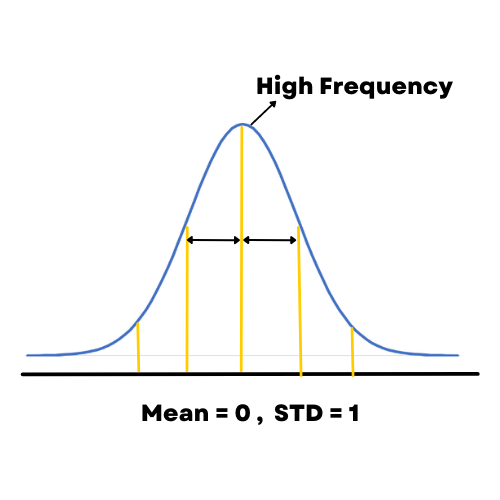## Method 1: Implementation in pandas [Z-Score]

To standardize the data in pandas, Z-Score is a very popular method in pandas that is used to standardize the data. Z-Score will tell us how many standard deviations away a value is from the mean. when we standardize the data the data will be changed into a specific form where the graph of its frequency will form a bell curve. The formula to convert the data is,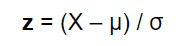Syntax:

df[‘column’] =( df[‘column’] – df[‘column’].mean() ) / df[‘column’].std()

In this method, we are going to standardize the first column of the data set using pandas built-in functions mean() and std() which will give the mean and standard deviations of the column data. So that using a simple calculation of subtracting the element with its mean and dividing them with the standard deviation will give us the z-score of the data which is the standardized data.

Data frame in use: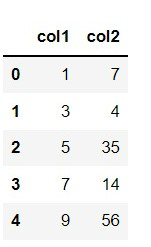Example: Standardizing data

## Python3

 `# Importing the library ` `import` `pandas as pd ` ` `  `# Creating the data frame ` `details ``=` `{ ` `    ``'col1'``: [``1``, ``3``, ``5``, ``7``, ``9``], ` `    ``'col2'``: [``7``, ``4``, ``35``, ``14``, ``56``] ` `} ` ` `  `# creating a Dataframe object ` `df ``=` `pd.DataFrame(details) ` ` `  `# Z-Score using pandas ` `df[``'col1'``] ``=` `(df[``'col1'``] ``-` `df[``'col1'``].mean()) ``/` `df[``'col1'``].std() `

Output: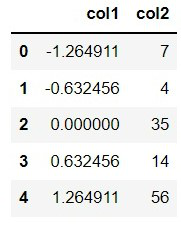## Method 2 : Using scipy.stats()

Scipy is a scientific calculations library. It can single-handedly handle any complex maths calculations. Like every calculation scipy also can handle statistic calculations so we can find the z score of any column with just a line of code.

Syntax:

scipy.stats.zscore( df[‘column’] )

Now we are going to standardize the second column of our data by finding the z score using scipy.stats.zscore() we just need to mention the column and the library will take care of everything.

Example: Standardizing values

## Python

 `# Importing the library ` `import` `pandas as pd ` `import` `scipy ` `from` `scipy ``import` `stats ` ` `  ` `  `# Creating the data frame ` `details ``=` `{ ` `    ``'col1'``: [``1``, ``3``, ``5``, ``7``, ``9``], ` `    ``'col2'``: [``7``, ``4``, ``35``, ``14``, ``56``] ` `} ` ` `  `# creating a Dataframe object ` `df ``=` `pd.DataFrame(details) ` ` `  `# Z-Score using scipy ` `df[``'col2'``] ``=` `stats.zscore(df[``'col2'``]) `

Output: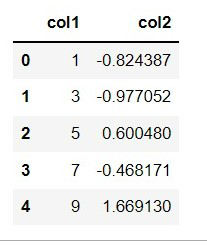## Method 3: Using sci-kit learn Standard scaler

Sci-kit earn is a machine learning and model building library. We can perform many operations in this library like preprocessing, Analyzing, and also model building for all kinds of machine learning like supervised, and Unsupervised learning problems. In this library, a preprocessing method called standardscaler() is used for standardizing the data.

Syntax:

scaler = StandardScaler()

df = scaler.fit_transform(df)

In this example, we are going to transform the whole data into a standardized form. To do that we first need to create a standardscaler() object and then fit and transform the data.

Example: Standardizing values

## Python

 `# Importing the library ` `import` `pandas as pd ` `from` `sklearn.preprocessing ``import` `StandardScaler ` ` `  ` `  `# Creating the data frame ` `details ``=` `{ ` `    ``'col1'``: [``1``, ``3``, ``5``, ``7``, ``9``], ` `    ``'col2'``: [``7``, ``4``, ``35``, ``14``, ``56``] ` `} ` ` `  `# creating a Dataframe object ` `df ``=` `pd.DataFrame(details) ` ` `  `# define standard scaler ` `scaler ``=` `StandardScaler() ` ` `  `# transform data ` `df ``=` `scaler.fit_transform(df) `

Output: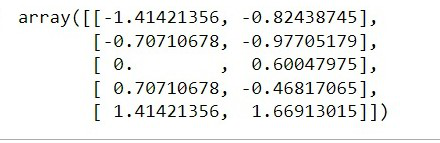My Personal Notes arrow_drop_up
Recommended Articles
Page :